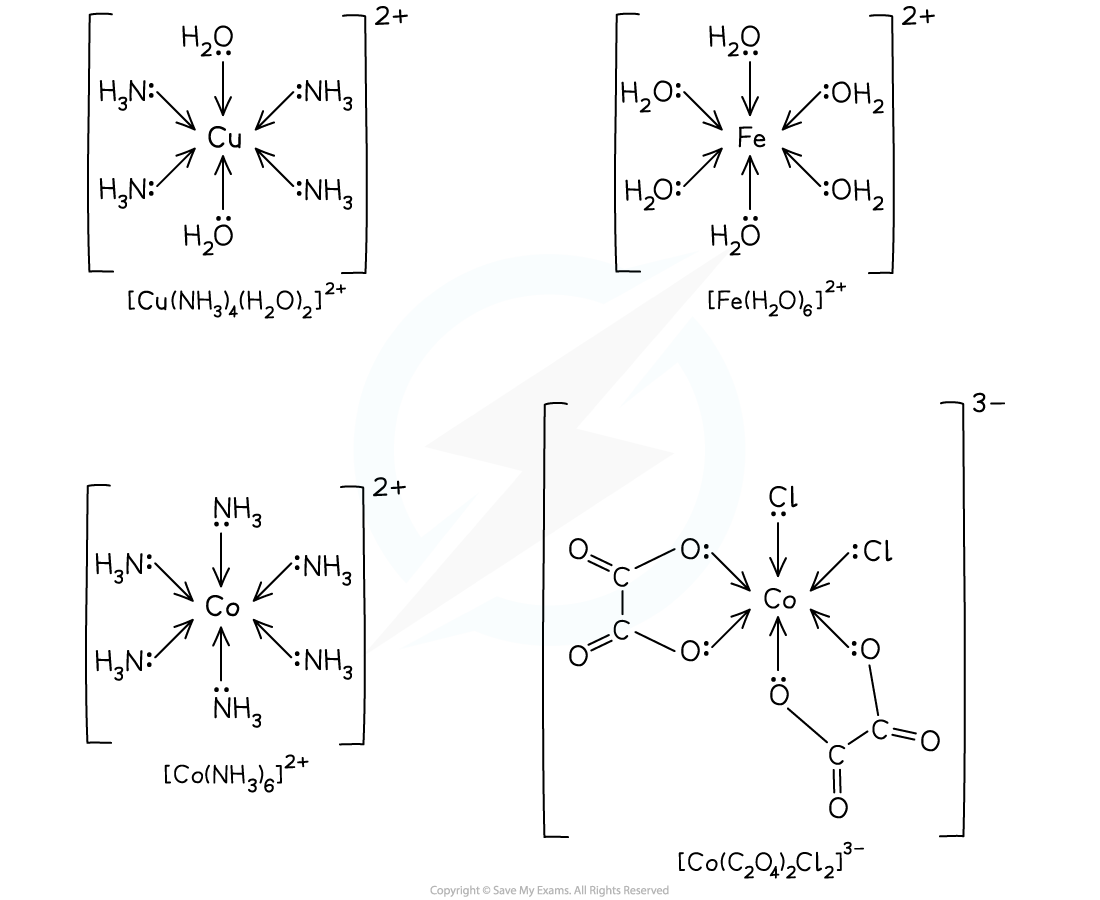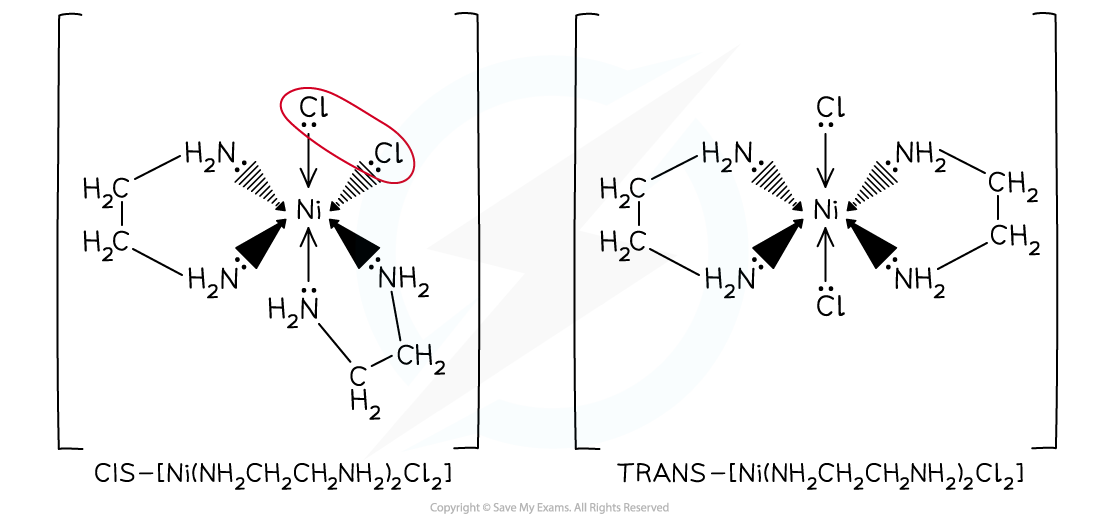# AQA A Level Chemistry复习笔记 6.2.3 Shapes of Complex Ions

### Shapes of Complex Ions

• Depending on the size of the ligands and the number of dative bonds to the central metal ion, transition element complexes have different geometries
• Dative bonds can also be referred to as coordinate bonds, especially when discussing the geometry of a complex

#### Linear

• Central metal atoms or ions with two coordinate bonds form linear complexes
• The bond angles in these complexes are 180o
• The most common examples are a copper (I) ion, (Cu+), or a silver (I) ion, (Ag+), as the central metal ion with two coordinate bonds formed to two ammonia ligandsExamples of a linear complex

• The second example is the diamminesilver(I) ion, [Ag(NH₃)₂]⁺, which is present in Tollens' reagent
• Tollens' reagent is used to test for the aldehyde functional group in organic molecules
• In the test, the silver(I) ion is reduced to silver atoms that produce a characteristic silver mirror on the test tube walls

#### Tetrahedral

• When there are four coordinate bonds the complexes often have a tetrahedral shape
• Complexes with four chloride ions most commonly adopt this geometry
• Chloride ligands are large, so only four will fit around the central metal ion

• The bond angles in tetrahedral complexes are 109.5oExample of a tetrahedral complex

#### Square planar

• Sometimes, complexes with four coordinate bonds may adopt a square planar geometry instead of a tetrahedral one
• Cyanide ions (CN-) are the most common ligands to adopt this geometry
• An example of a square planar complex is cisplatin

• The bond angles in a square planar complex are 90oCisplatin is an example of a square planar complex

#### Octahedral

• Octahedral complexes are formed when a central metal atom or ion forms six coordinate bonds
• This could be six coordinate bonds with six small, monodentate ligands
• Examples of such ligands are water and ammonia molecules and hydroxide and thiocyanate ions

• It could be six coordinate bonds with three bidentate ligands
• Each bidentate ligand will form two coordinate bonds, meaning six coordinate bonds in total
• Examples of these ligands are 1,2-diaminoethane and the ethanedioate ion

• It could be six coordinate bonds with one multidentate ligand
• The multidentate ligand, for example EDTA4-, forms all six coordinate bonds

• The bond angles in an octahedral complex are 90oExamples of octahedral complexes

### Isomerism in Complex Ions

• Transition element complexes can exhibit stereoisomerism

#### Geometrical (cis-trans) isomerism

• Even though transition element complexes do not have a double bond, they can still have geometrical isomers
• Square planar and octahedral complexes with two pairs of different ligands exhibit cis-trans isomerism (this is a special case of E-Z isomerism)
• An example of a square planar complex with two pairs of ligands is the anti-cancer drug cis-platin
• Whereas cis-platin has beneficial medical effects by binding to DNA in cancer cells, trans-platin cannot be used in cancer treatmentCis-platin (the Z-isomer) and trans-platin (the E-isomer) is an example of a square planar transition element complex that exhibits geometrical isomerism

• As long as a complex ion has two ligands attached to it that are different to the rest, then the complex can display geometric isomerism
• Examples of octahedral complexes that exhibit geometrical isomerism are the [Cu(NH3)4(H2O)2]2+ and [Ni(H2NCH2CH2NH2)2Cl2]2+ complexes
• [Ni(H2NCH2CH2NH2)2Cl2]2+ can also be written as [Ni(en)2Cl2]2+

• Like in the square planar complexes, if the two ‘different’ ligands are adjacent (next) to each other then that is the ‘cis’ isomer, and if the two ‘different’ ligands are opposite each other then this is the ‘trans’ isomer
• In [Cu(NH3)4(H2O)2]2+, the two water ligands are adjacent to each other in the cis isomer and are opposite each other in the trans isomerOctahedral transition metal complexes exhibiting geometrical isomerism

#### Optical isomerism

• Octahedral complexes with bidentate ligands also have optical isomers
• This means that the two forms are non-superimposable mirror images of each other
• They have no plane of symmetry, and one image cannot be placed directly on top of the other

• The optical isomers only differ in their ability to rotate the plane of polarised light in opposite directions
• Examples of octahedral complexes that have optical isomers are the [Ni(H2NCH2CH2NH2)3]2+and [Ni(H2NCH2CH2NH2)2(H2O)2]2+ complexes
• The ligand H2NCH2CH2NH2 can also be written as ‘en’ insteadOctahedral transition metal complexes exhibiting optical isomerism

#### Drawing stereochemical formulae

• Chemists use a convention of wedge drawings to represent three dimensional molecules
• The convention is that
• a solid line is a bond in the same plane as the paper
• a dotted line is a bond receding behind the plane of the paper(this can also be hatched or shaded wedges)
• a solid wedge is a bond coming out of the paperFour main shapes of transition metal complexes using stereochemical formulae